Students can Download Maths Chapter 4 Geometry Ex 4.1 Questions and Answers, Notes Pdf, Samacheer Kalvi 7th Maths Book Solutions Guide Pdf helps you to revise the complete Tamilnadu State Board New Syllabus and score more marks in your examinations.

## Tamilnadu Samacheer Kalvi 7th Maths Solutions Term 2 Chapter 4 Geometry Ex 4.1

Question 1.
Can 30°, 60° and 90° be the angles of a triangle?
Solution:
Given angles 30°, 60° and 90°
Sum of the angles = 30° + 60° + 90° = 180°
∴ The given angles form a triangle.

Question 2.
Can you draw a triangle with 25°, 65° and 80° as angles?
Solution:
Given angle 25°, 65° and 80°.
Sum of the angles = 25° + 65° + 80° = 170° ≠ 180
∴ We cannot draw a triangle with these measures.Question 3.
In each of the following triangles, find the value of x.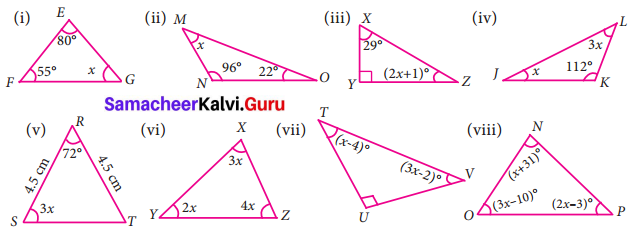Solution:
(i) Let ∠G = x
By angle sum property we know that,
∠E + ∠F + ∠G = 180°
80° + 55° + x = 180°
135° + x = 180°
x = 45°

(ii) Let ∠M = x
By angle sum property of triangles we have
∠M + ∠M + ∠O = 180°
x + 96° + 22° = 180°
x + 118° = 180°
X = 180° – 118° = 620

(iii) Let ∠Z = (2x + 1)° and ∠Y = 90°
By the sum property of triangles we have
∠x + ∠y + ∠z = 180°
29° + 90° + (2x + 1)° = 180°
119° + (2x + 1)° = 180°
(2x + 1)° = 180° – 119°
2x + 1° = 61°
2x = 61° – 1°
2x = 60°
x = $$\frac{60^{\circ}}{2}$$
x = 30°

(iv) Let ∠J = x and ∠L – 3x.
By angle sum property of triangles we have
∠J + ∠K + ∠L = 180°
x + 112° + 3x = 180°
4x = 180° – 112°
x = 68°
x = $$\frac{68^{\circ}}{4}$$
x = 17°

(v) Let ∠S = 3x°
Given $$\overline{\mathrm{RS}}$$ = Given $$\overline{\mathrm{RT}}$$ = 4.5 cm
Given ∠S = ∠T = 3x° [∵ Angles opposite to equal sides are equal]
By angle sum property of a triangle we have,
∠R + ∠S + ∠T = 180°
72° + 3x + 3x = 180°
72° + 6x = 180°
x = $$\frac{108^{\circ}}{6}$$
x = 18°

(vi) Given ∠X = 3x; ∠Y = 2x; ∠Z = ∠4x
By angle sum property of a triangle we have
∠X + ∠Y + ∠Z = 180°
3x + 2x + 4x = 180°
∴ 9x = 180°
x = $$\frac{180^{\circ}}{9}$$ = 20°

(vii) Given ∠T = (x – 4)°
∠U = 90°
∠V = (3x – 2)°
By angle sum property of a triang we have
∠T + ∠U + ∠V = 180°
(x – 4)° + 90° + (3x – 2)° = 180°
x – 4° + 90° + 3x – 2° = 180°
x + 3x + 90° – 4° – 2° = 180°
4x + 84° = 180°
4x = 180° – 84°
4x = 96°
x = $$\frac{96^{\circ}}{4}$$ = 24°
x = 24°

(viii) Given ∠N = (x + 31)°
∠O = (3x – 10)°
∠P = (2x – 3)°
By angle sum property of a triangle we have
∠N + ∠O + ∠P = O
(x + 31)° + (3x – 10)° + (2x – 3)° = 180°
x + 31°+ 3x – 10° + 2x – 3° = 180°
x + 3x + 2x + 31° – 10° – 3° = 180°
6x + 18° = 180°
6x = 180° + 18°
6x = 162°
x = $$\frac{162^{\circ}}{6}$$ = 27°
x = 27°Question 4.
Two line segments $$\overline{A D}$$ and $$\overline{B C}$$ intersect at O. Joining $$\overline{A B}$$ and $$\overline{D C}$$ we get two triangles, ∆AOB and ∆DOC as shown in the figure. Find the ∠A and ∠B.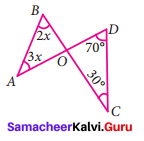Solution:
In ∆AOB and ∆DOC,
∠AOB = ∠DOC [∵ Vertically opposite angles are equal]
Let ∠AOB = ∠DOC = y
By angle sum property of a triangle we have
∠A + ∠B + ∠AOB = ∠D + ∠C + ∠DOC = 180°
3x + 2x + y = 70° + 30° + y = 180°
5x + y = 100° + y = 180°
Here 5x + y = 100° + y
5x = 100° + y – y
5x = 100°
x = $$\frac{100^{\circ}}{5}$$ = 20°
∠A = 3x = 3 × 20 = 60°
∠B = 2x = 2 × 20 = 40°
∠A = 60°
∠B = 40°

Question 5.
Observe the figure and find the value of
∠A + ∠N + ∠G + ∠L + ∠E + ∠S.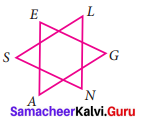Solution:
In the figure we have two triangles namely ∆AGE and ∆NLS.
By angle sum property of triangles,
Sum of angles of ∆AGE = ∠A + ∠G + ∠E = 180° …(1)
Also sum of angles of ∆NLS = ∠N + ∠L + ∠S = 180° … (2)
(1) + (2) ∠A + ∠G + ∠E + ∠N + ∠L + ∠S = 180° + 180°
i.e., ∠A + ∠N + ∠G + ∠L + ∠E + ∠S = 360°Question 6.
If the three angles of a triangle are in the ratio 3 : 5 : 4, then find them.
Solution:
Given three angles of the triangles are in the ratio 3 : 5 : 4.
Let the three angle be 3x, 5x and 4x.
By angle sum property of a triangle, we have
3x + 5x + 4x = 180°
12x = 180°
x = $$\frac{180^{\circ}}{12}$$
x = 15°
∴ The angle are 3x = 3 × 15° = 45°
5x = 5 × 15° = 75°
4x = 4 × 15° = 60°
Three angles of the triangle are 45°, 75°, 60°

Question 7.
In ∆RST, ∠S is 10° greater than ∠R and ∠T is 5° less than ∠S , find the three angles of the triangle.
Solution:
In ∆RST. Let ∠R = x.
Then given S is ∠10° greater than ∠R
∴ ∠S = x + 10°
Also given ∠T is 5° less then ∠S.
So ∠T = ∠S – 5° = (x + 10)° – 5° = x + 10° – 5°
By angle sum property of triangles, sum of three angles = 180°.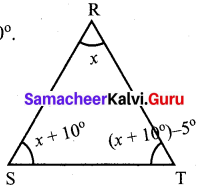∠R + ∠S + ∠T = 180°
x + x + 10° + x + 5° = 180°
3x + 15° = 180°
3x = 180° – 15°
x = $$\frac{165^{\circ}}{3}$$ = 55°
∠R = x = 55°
∠S = x + 10° = 55° + 10° = 65°
∠T = x + 5° = 55° + 5° = 60°
∴ ∠R = 55°
∠S = 65°
∠T = 60°

Question 8.
In ∆ABC , if ∠B is 3 times ∠A and ∠C is 2 times ∠A, then find the angles.
Solution:
In ABC, Let ∠A = x,
then ∠B = 3 times ∠A = 3x
∠C = 2 times ∠A = 2x
By angle sum property of a triangles,
Sum of three angles of ∆ABC =180°.
∠A + ∠B + ∠C = 180
x + 3x + 2x = 180°
x (1 + 3 + 2) = 180°
6x = 180°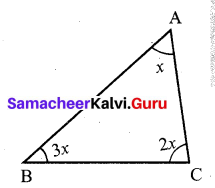x = $$\frac{180^{\circ}}{6}$$ = 30°
∠A = x = 30°
∠B = 3x = 3 × 30° = 90°
∠C = 2x = 2 × 30° = 60°
∴ ∠A = 30°
∠B = 90°
∠C = 60°Question 9.
In ∆XYZ, if ∠X : ∠Z is 5 : 4 and ∠Y = 72°. Find ∠X and ∠Z.
Solution:
Given in ∆XYZ, ∠X : ∠Z = 5 : 4
Let ∠X = 5x; and ∠Z = 4x given ∠Y = 72°
By the angle sum property of triangles sum of three angles of a triangles is 180°.
∠X + ∠Y + ∠Z = 180°
5x + 72 + 4x = 180°
5x + 4x = 180° – 72°
9x = 108°
x = $$\frac{108^{\circ}}{9}$$ = 12°
∠X = 5x = 5 × 12° = 60°
∠Z = 4x = 4 × 12° = 48°
∴ ∠X = 60°
∠Z = 48°

Question 10.
In a right angled triangle ABC, ∠B is right angle, ∠A is x + 1 and ∠C is 2x + 5. Find ∠A and ∠C.
Solution:
Given in ∆ABC ∠B = 90°
∠A = x + 1
∠B = 2x + 5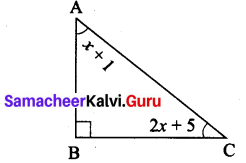By angle sum property of triangles
Sum of three angles of ∆ABC = 180°
∠A + ∠B + ∠C = 180°
(x + 1) + 90° + (2x + 5) = 180°
x + 2x + 1° + 90° + 5° = 180°
3x + 96° = 180°
3x = 180° – 96° = 84°
x = $$\frac{84^{\circ}}{3}$$ = 28°
∠A = x + 1 = 28 + 1 = 29
∠C = 2x + 5 = 2 (28) + 5 = 56 + 5 = 61
∴ ∠A = 29°
∠C = 61°Question 11.
In a right angled triangle MNO, ∠N = 90°, MO is extended to P. If ∠NOP = 128°, find the other two angles of ∆MNO.
Solution:
Given ∠N = 90°
MO is extended to P, the exterior angle ∠NOP = 128°
Exterior angle is equal to the sum of interior opposite angles.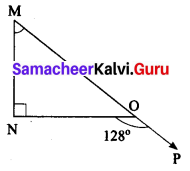∴ ∠M + ∠N = 128°
∠M + 90° = 128°
∠M = 128° – 90°
∠M = 38°
By angle sum property of triangles,
∴ ∠M + ∠N + ∠O = 180°
38° + 90° + ∠O = 180°
∠O = 180° – 128°
∠O = 52°
∴ ∠M = 38° and ∠O = 52°

Question 12.
Find the value of x in each of the given triangles.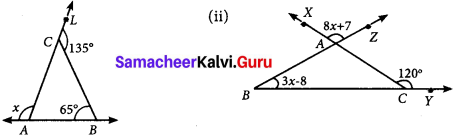Solution:
(i) In ∆ABC, given B = 65°,
AC is extended to L, the exterior angle at C, ∠BCL = 135°
Exterior angle is equal to the sum of opposite interior angles.
∠A + ∠B = ∠BCL
∠A + 65° = 135°
∠A = 135° – 65°
∴ ∠A = 70°
x + ∠A = 180° [∵ linear pair]
x + 70° = 180° [∵ ∠A = 70°]
x = 180° – 70°
∴ x = 110°

(ii) In ∆ABC, given B = 3x – 8°
∠XAZ = ∠BAC [∵ vertically opposite angles]
8x + 7 + ∠BAC
i.e., In ∆ABC, ∠A = 8x + 7
Exterior angle ∠XCY = 120°
Exterior angle is equal to the sum of the interior opposite angles.
∠A + ∠B = 120°
8x + 7 + 3x – 8 = 120°
8x + 3x = 120° + 8 – 7
11x = 121°
x = $$\frac{121^{\circ}}{11}$$ = 11°

Question 13.
In ∆LMN, MN is extended to O. If ∠MLN = 100 – x, ∠LMN = 2x and ∠LNO = 6x – 5, find the value of x.
Solution:
Exterior angle is equal to the sum of the opposite interior angles.
∠LNO = ∠MLN + ∠LMN6x – 5 = 100° – x + 2x
6x – 5 + x – 2x = 100°
6x + x – 2x = 100° + 5°
5x = 105°
x = $$\frac{105^{\circ}}{5}$$ = 21°
x = 21°Question 14.
Using the given figure find the value of x.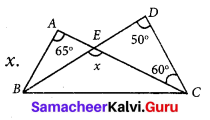Solution:
In ∆EDC, side DE is extended to B, to form the exterior angle ∠CEB = x.
We know that the exterior angle is equal to the sum of the opposite interior angles
∠CEB = ∠CDE + ∠ECD
x = 50° + 60°
x = 110°

Question 15.
Using the diagram find the value of x.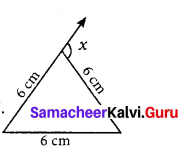Solution:
Given triangle is an equilateral triangle as the three sides are equal. For an equilateral triangle all three angles are equal and is equal to 60° Also exterior angle is equal to sum of opposite interior angles.
x = 60° + 60°.
x = 120°

Objective Type Questions

Question 16.
The angles of a triangle are in the ratio 2:3:4. Then the angles are
(i) 20,30,40
(ii) 40, 60, 80
(iii) 80, 20, 80
(iv) 10, 15, 20
(ii) 40, 60, 80

Question 17.
One of the angles of a triangle is 65°. If the difference of the other two angles is 45°, then the two angles are
(i) 85°, 40°
(ii) 70°, 25°
(iii) 80°, 35°
(iv) 80° , 135°
(iii) 80°,35°Question 18.
In the given figure, AB is parallel to CD. Then the value of b is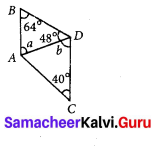(i) 112°
(ii) 68°
(iii) 102°
(iv) 62° A
(ii) 68°

Question 19.
In the given figure, which of the following statement is true?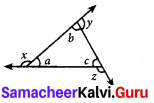(i) x + y + z = 180°
(ii) x + y + z = a + b + c
(iii) x + y + z = 2(a + b + c)
(iv) x + y + z = 3(a + b + c)
Ans :
(iii) x + y + z = 2(a + b + c)]

Question 20.
An exterior angle of a triangle is 70° and two interior opposite angles are equal. Then measure of each of these angle will be
(i) 110°
(ii) 120°
(iii) 35°
(iv) 60°
(iii) 35°Question 21.
In a ∆ABC, AB = AC. The value of x is _____.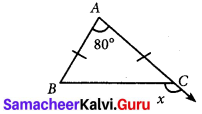(i) 80°
(ii) 100°
(iii) 130°
(iv) 120°